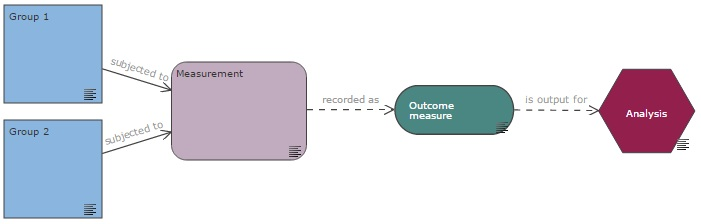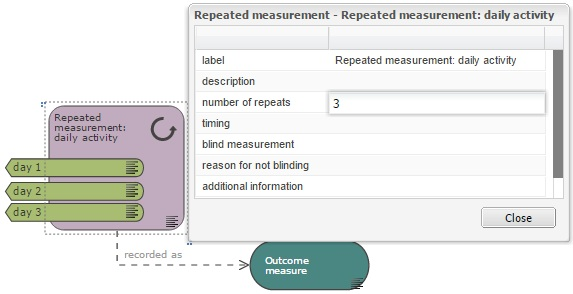# Measurement

A measurement is a process which a group of animals (or experimental units) is subjected to, in order to collect data; it is recorded as at least one outcome measure.

Content:

In the EDA, three different types of nodes are used to represent data collection. The measurement and repeated measurement nodes are used to describe the process; these nodes have to be used in conjunction with an outcome measure node. Additional pre-analysis processing is indicated with a data transformation node.

## Measurement nodes

Every experiment diagram should feature a measurement node as all groups should at one point be subjected to a measurement which is recorded as one or several outcome measures. The outcome measure (dependent variable) is then used as the output for the analysis.If time is a variable in the experiment (either a nuisance variable or a variable of interest), measurements nodes should be tagged with the appropriate variable categories. For example in Example 2, the test period is a nuisance variable and measurement nodes are tagged with the categories ‘period 1’ and ‘period 2’.

The measurement node refers to a single measurement. If the same measurement is repeated multiple times consecutively, for example over several days, and the animals are not subjected to any other intervention during that period, a repeated measurement node can be used to simplify the diagram (see Example 3) and the number of times this measurement is repeated should be indicated in the properties of the repeated measurement node. If time is an independent variable in the experiment, the repeated measurement node should be tagged with all the variable categories nodes of all time points represented by the repeated measurement node, as shown in Example 3 and the image below.Additional information can be provided in the properties of either measurement nodes. Timing refers for example to the time of the day at which the measurement was taken, the time relative to the start of the experiment or to a specific intervention, or the length of time each measurement takes, if it is relevant. For a repeated measurement, it can also refer to the frequency at which measurements are taken.

The blinding status during the measurement should be indicated; concealment of the group allocation during result assessment can be achieved with the help of a colleague who can code the animals (or the units of measurement) so that the investigator conducting the measurement does not know what treatment animals have received or what group they belong to. Alternatively, the investigator can code the animals and let a colleague do the measurements.

If a measurement is conducted without blinding and the investigator is aware of the group allocation while measuring the outcome, it should be specified along with the reason why blinding was not possible. For example, animals in different treatment groups have different coat colours. If blinding is not possible at the measurement stage, it is especially important to ensure that subjective bias is reduced at every other stage of the experiment, with allocation concealment and blinded analysis of the data (see blinding section).

## Outcome measure

A measurement is recorded as an outcome measure (also known as dependent variable). A measurement can be recorded as more than one outcome measure. For example, if activity is measured, one outcome measure could be the time spent walking and the other the distance travelled. Each can be fully defined in the properties of their respective node.

The measurement and outcome measure nodes can either be connected with a ‘recorded as’ link or, to simplify the diagram, a measurement node can be ‘tagged’ with outcome measures nodes, as shown below in the image on the right.### Continuous or categorical outcome measure

Outcome measures can be categorical or continuous; this needs be indicated in the properties of the outcome measure node so that the system can recommend an adequate analysis recommendation.

Continuous data are sometimes referred to as quantitative data and are measured on a numerical scale. Continuous measures include truly continuous data but also discrete data. Examples of true continuous data include bodyweight, body temperature, blood / CSF concentration or time to event, while examples of discrete data include litter size, number of correct response or clinical score.

Categorical responses are measured on a non-numerical scale; they can be ordinal (e.g. severity score: mild/moderate/severe), nominal (e.g. animal’s reaction: left/middle/right arm maze) or binary (e.g. disease state: present/absent).

Continuous responses may take longer to measure but they contain more information. If possible, it is preferable to measure a continuous rather than categorical response because continuous data can be analysed using the parametric analyses, which have higher power; this reduces the sample size needed.

### Linking the variability of the outcome measure to the sample size

The anticipated standard deviation can be indicated within the properties of the outcome measure node. This is estimated based on pilot study data, prior experience, or the literature as appropriate. The standard deviation of the primary outcome measure is used to estimate the sample size needed for the experiment, based on a power calculation.

The primary outcome measure should be identified in the planning stage of the experiment; it is the outcome of greatest importance, which will answer the main experimental question. The number of animals in the experiment is determined by the power needed to detect a difference in the primary outcome measure.

## Data transformation

If the outcome measure is transformed, this can be indicated by connecting it to a transformation node. Data transformation nodes are outcome-specific; two different outcome measures cannot be connected to the same data transformation node even if both outcomes are transformed the same way. The data transformation produces the output which is used in the analysis.The data transformation node is used to indicate two different types of transformation: summary measures and transformation to normalise the data.

### Summary measures

Summary measures can be used when animals (or experimental units) are measured repeatedly, this could be several time points or several brain regions for example. In Example 3, the variable ‘time of measurement’ is not included in the analysis. For each animal, a summary measure of all time points – the area under the curve (AUC) – is used instead. This simplifies the analysis as it reduces the number of factors of interest.

Examples of summary measures include AUC, Tmax, Cmax, ED50, average per animal or percentage of baseline. Whether the outcome measure is expressed as the raw values or as a summary measure should be indicated in the properties of the data transformation node. In the absence of a data transformation node, it is assumed that raw values are used in the analysis.

### Transformation to normalise the data

If the response, or more precisely the residuals from the analysis, are not normally distributed – whether this is anticipated at the planning stage, or this is decided once the data is collected – it can usually be normalised to enable the use of parametric tests.

Common transformations include logarithmic, square root and arcsine; this is described more extensively in the analysis section.